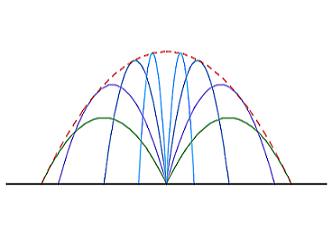# The Orbits' Envelope of the ProjectilesSuppose at initial height $0$, one casts a projectile into the air with constant initial velocity $v$ but different elevation angles in the same vertical plane. The area of the set of all points that can be reached by the projectile is $A=\alpha\frac {v^4}{g^2}.$ What is the value of positive number $\alpha?$

Neglect air resistance.

×